3.6 Binary Search Tree in Array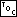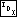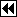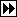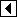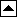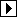Binary search is pretty fast. Suppose that we wish to speed it up anyhow. Then, the obvious speed-up targets in <Binary search of ordered array 21> above are the while condition and the calculations determining values of i, min, and max. If we could eliminate these, we'd have an incrementally faster technique, all else being equal. And, as it turns out, we can eliminate both of them, the former by use of a sentinel and the latter by precalculation.

Let's consider precalculating i, min, and max first. Think about the nature of the choices that binary search makes at each step. Specifically, in <Binary search of ordered array 21> above, consider the dependence of min and max upon i. Is it ever possible for min and max to have different values for the same i and n?

The answer is no. For any given i and n, min and max are fixed. This is important because it means that we can represent the entire “state” of a binary search of an n-element array by the single variable i. In other words, if we know i and n, we know all the choices that have been made to this point and we know the two possible choices of i for the next step.

This is the key insight in eliminating calculations. We can use an array in which the items are labeled with the next two possible choices.

An example is indicated. Let's continue with our example of an array containing the 16 integers 100 to 115. We define an entry in the array to contain the item value and the array index of the item to examine next for search values smaller and larger than the item:

```22. <Binary search tree entry 22> =
/* One entry in a binary search tree stored in an array. */
struct binary_tree_entry   {
int value;          /* This item in the binary search tree. */
int smaller;        /* Array index of next item for smaller targets. */
int larger;         /* Array index of next item for larger targets. */
};
```

This code is included in 617.

Of course, it's necessary to fill in the values for smaller and larger. A few moments' reflection should allow you to figure out one method for doing so. Here's the full array, for reference:

```const struct binary_tree_entry bins =   {
{100, 15, 15},     {101, 0, 2},     {102, 15, 15},     {103, 1, 5},     {104, 15, 15},
{105, 4, 6},     {106, 15, 15},     {107, 3, 11},     {108, 15, 15},     {109, 8, 10},
{110, 15, 15},     {111, 9, 13},     {112, 15, 15},     {113, 12, 14},     {114, 15, 15},
{0, 0, 0},
};
```

For now, consider only bins[]'s first 15 rows. Within these rows, the first column is value, the item value, and the second and third columns are smaller and larger, respectively. Values 0 through 14 for smaller and larger indicate the index of the next element of bins[] to examine. Value 15 indicates “element not found”. Element array is not used for storing data.

Try searching for key == 110 in bins[], starting from element 7, the midpoint:

1. i == 7: 110 > bins[i].value == 107, so let i = bins[i].larger, or 11.
2. i == 11: 110 < bins[i].value == 111, so let i = bins[i].smaller, or 10.
3. i == 10: 110 == bins[i].value == 110, so we're done.

We can implement this search in C code. The function uses the common C idiom of writing for (;;) for an “infinite” loop:

```23. <Search of binary search tree stored as array 23> =
/* Returns i such that array[i].value == key,    or -1 if key is not in array[].
array[] is an array of n elements forming a binary search tree,
with its root at array[n / 2],    and space for an (n + 1)th value at the end. */
int binary_search_tree_array (struct binary_tree_entry array[], int n,                           int key) {
int i = n / 2;

array[n].value = key;
for (;;)
if (key > array[i].value)       i = array[i].larger;
else if (key < array[i].value)       i = array[i].smaller;
else       return i != n ? i : -1;
}
```

This code is included in 617.

Examination of the code above should reveal the purpose of bins. It is used as a sentinel value, allowing the search to always terminate without the use of an extra test on each loop iteration.

The result of augmenting binary search with “pointer” values like smaller and larger is called a binary search tree (see binary search tree).

Exercises:

1. Write a function to automatically initialize smaller and larger within bins[]. [answer]

2. Write a simple automatic test program for binary_search_tree_array(). Let the user specify the size of the array to test on the command line. You may want to use your results from the previous exercise. [answer]